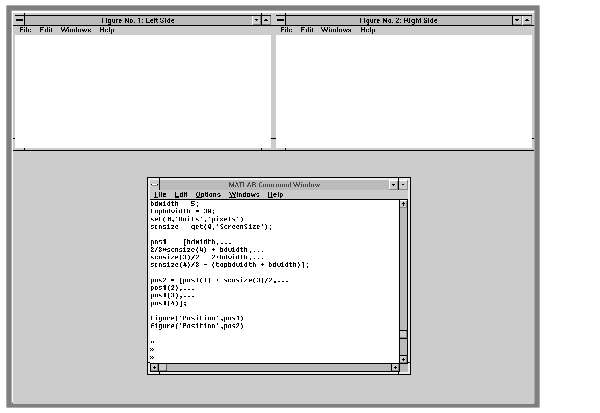GraphicsExample -- Specifying Figure Position

Suppose you want to define two figure windows that occupy the upper third of the computer screen (e.g., one for uicontrols and the other to display data). To position the windows precisely, you must consider the window borders when calculating the size and offsets to specify for the `Position` properties.

1. The figure `Position` property does not include the window borders, so this example uses a width of 5 pixels on the sides and bottom and 30 pixels on the top.
• ```bdwidth = 5;
topbdwidth = 30;
```
2. Ensure root units are pixels and get the size of the screen:
• ```set(0,'Units','pixels')
scnsize = get(0,'ScreenSize');
```
3. Define the size and location of the figures:
• ```pos1  = [bdwidth,...
2/3*scnsize(4) + bdwidth,...
scnsize(3)/2 - 2*bdwidth,...
scnsize(4)/3 - (topbdwidth + bdwidth)];
pos2 = [pos1(1) + scnsize(3)/2,...
pos1(2),...
pos1(3),...
pos1(4)];
```
4. Create the figures:
• ```figure('Position',pos1)
figure('Position',pos2)
```

The two figures now occupy the top third of the screen.Positioning Figures Figure Colormaps -- The Colormap Property© 1994-2005 The MathWorks, Inc.